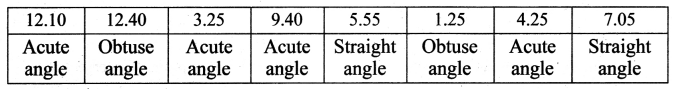## Tamilnadu Samacheer Kalvi 6th Maths Solutions Term 1 Chapter 4 Geometry Intext Questions

Try These (Textbook Page No. 80, 81)

Question 1.
Name all the line segments.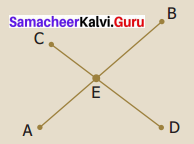Solution:
$$\overline{\mathrm{AB}}, \overline{\mathrm{AE}}, \overline{\mathrm{EB}}, \overline{\mathrm{CD}}, \overline{\mathrm{CE}} \text { and } \overline{\mathrm{ED}}$$

Question 2.
If AB = 5 cm, say which of the following measures are correct in fig 4.9.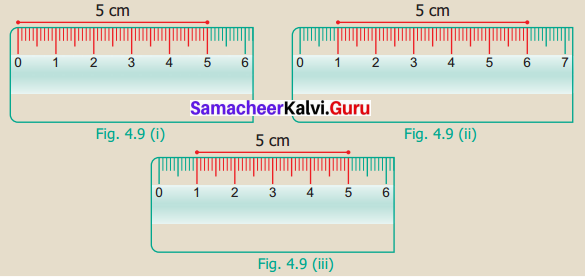Solution:
Fig 4.9(i) and fig 4.9(ii) measures are correct.Try These (Textbook Page No. 85)

Question 1.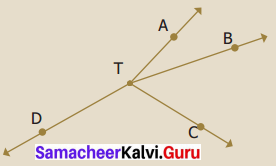1. Name the rays in the given figure.
2. What is the common point of all these rays?
Solution:
1. $$\overrightarrow{\mathrm{TA}}, \overrightarrow{\mathrm{TB}}, \overrightarrow{\mathrm{TC}} \text { and } \overrightarrow{\mathrm{TD}}$$ are the rays given
2. Point T is the common point of all these rays.

Try These (Textbook Page No. 90, 95)

Question 1.
Which direction will you face if you start facing West and take three right turns clockwise?
Solution:
Will be facing South.

Question 2.
Which direction will you face if you start facing North and take two right turns anticlockwise?
Solution:
Will be facing South.

Question 3.
Adjust the hands of the clock for following time, note the angle made between the hour hand and the minute hand and write the type of angle.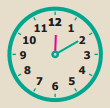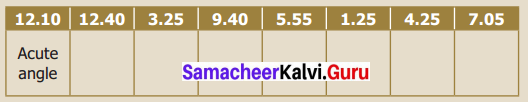Solution: